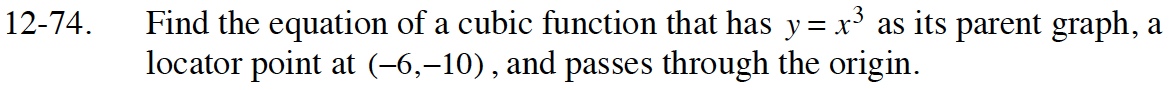Home > CCA2 > Chapter 12 > Lesson 12.1.4 > Problem12-74

12-74.

Find the equation of a cubic function that has y = x3 as its parent graph, a locator point at (−6, −10), and passes through the origin. Homework Help ✎Substitute the locator point (h, k) into the general equation
y = a(x − h)3 + k.

Use the known point, other than the locator point that lies on the graph, to solve
for the stretch factor, a. In this case, you are given the origin, (0, 0). Solve for a
and write the equation of the function.

0 = a(0 + 6) − 10